I.5.5

6/16/2021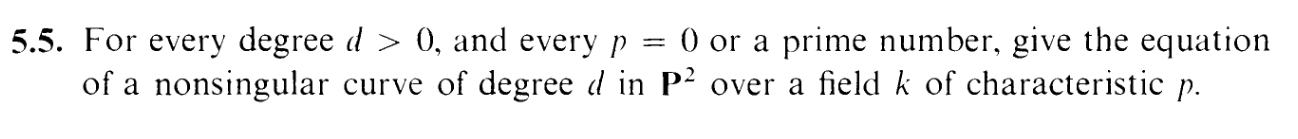Now, reader, shall I? that fossil sitting behind your cheekbone teases a hallway of intrigue within. Indeed, it is beckoning me to secrete my secrets into its corridors. Shall I run my tongue across that golden helix? Shall I nibble that lobule, dribble my tongue against that sensitive antitragus, or leave the whole artifact soaked in an indiscriminate slobber? Would it admit my silly trifles, my somber troubles? Would it submit to greedily brandished incisors? Would it forgive a lie; "Don't worry, I don't bite x3"? Would you be so kind as to lend it to me, today? I will give it back, I swear. Pretend my lips are the lips of a seashell, and my warm breath the sound of the ocean. For today I only have one little amulet to donate. One secret. One confession.... I fuked your mom lolololo jk but actually what I was gonna say is i looked at the solution for this exercise. *breathes sex noises into your ear*

I'm sorry, reader. Also, I'm skipping 5.4 because MY ABSTRACT ALGEBRA KNOWLEDGE IS SO FUCKING DEFICIENT LOL.

So, anyway, you may recall that we had a definition for nonsingularity for affine varieties: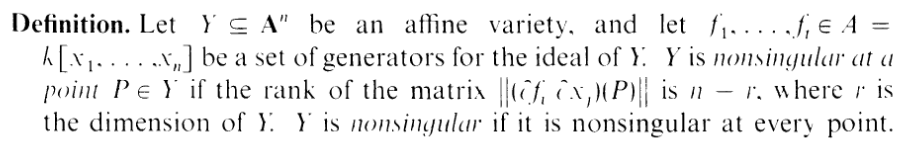In this exercise however, we're working with P2, so here's a generalization of the definition for more arbitrary varieties: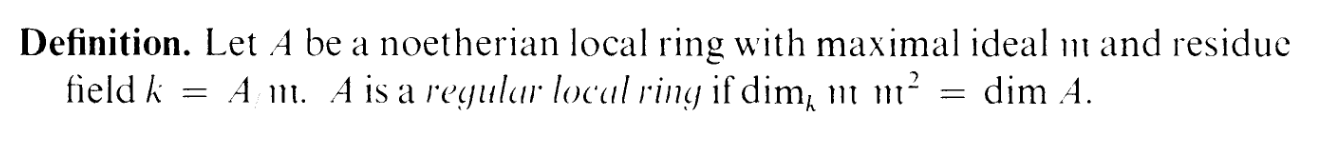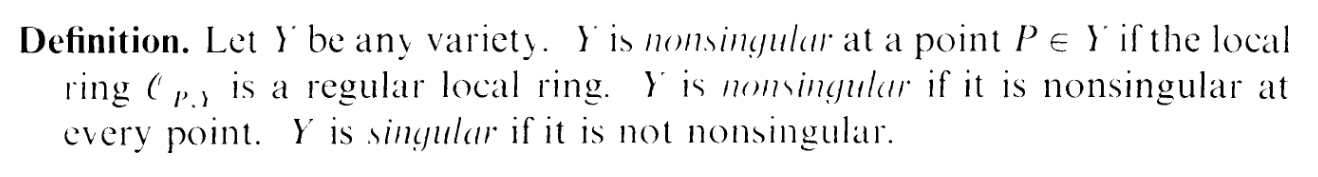Now, this definition is, as you can see, confusing as fuck. HOWEVER: Note that nonsingularity only depends on the local ring. So we can use open sets in P2 instead of P2 itself. I.e. we can cover P2 with A2s and just default to the more convenient definition with the Jacobian. So this problem is back to checking for nonzero Jacobians.

The solution says that

 xd + yd + zd = 0 (1)

works in almost all cases. Let's take the open set z0 (analogous argument for x0,y0. Then our curve equation turns into

 xd + yd + 1 = 0

in A2.

The Jacobian is then:

 J =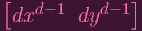Now the issue is that if chark divides d, then both components are 0, which is bad! So those are the cases for which this doesn't work, and we'll handle those later. However, in the cases that chark does not divide d, note that we would need x = 0 and y = 0 to make the Jacobian 0. But note that this isn't a point on the original curve, so the Jacobian is always nonzero.

Now we handle the chark|d case. The solution gives

 xyd-1 + yzd-1 + zxd-1 = 0

(yea, I wouldn't have thought of this on my own). So taking z0, we get

 xyd-1 + y + xd-1 = 0 (2)

And the Jacobian is

 J =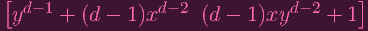=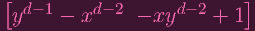(since chark|d)

So we need

 yd-1 = xd-2 (3) xyd-2 = 1 (4)

Multiplying both sides of the (4) by y and substuting (3) yields

 xyd-1 = y (5)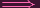xd-1 = y (6)

Now let's plug in (5) and (6) into the curve equation (2):

 xyd-1 + y + xd-1 = 0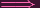y + y + y = 03y = 0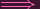y = 0

and thus (6) tells us that x = 0 as well. But this doesn't satisfy (4). Done.